### Approximate Methods: The Weighted Residuals Method

The statement of the equilibrium equations applied to a set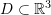is as follows. Assuming that at equilibrium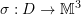is the symmetric Cauchy stress distribution on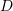and that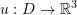is the displacement vector distribution and knowing the relationship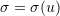, then the equilibrium equation seeks to find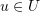such that the associatedsatisfies the equation: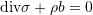where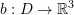is the body forces vector distribution on,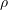is the mass density, and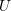is the space of all possible displacement functions applied to, i.e.,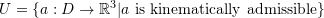. The term “Kinematically admissible” inindicates that the space of possible solutions must satisfy the boundary conditions imposed on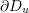(as stated below) and any differentiability constraints.

The boundary conditions for the equations of equilibrium are usually given on two parts of the boundary ofdenoted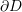. On the first part,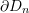, the external traction vectors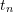are known so we have the boundary conditions forsince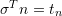(is the normal vector to the boundary). On the second part,, the displacement is given.

The weighted residuals method seeks an approximate solution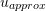with a particular form that has a finite number of unknown parameters. Sincedoes not necessarily satisfy the requirements for the problem, the corresponding stresses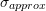would not satisfy equilibrium. The residuals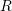are defined as the resulting values when the approximate solutions are substituted in the differential equation: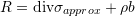Ifis the number of unknown parameters inthenweight functions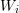can be chosen. For each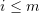, an integral form of the residuals is set to zero:

(1)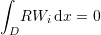The form ofshould be chosen satisfying the essential boundary conditions while the non-essential boundary conditions can be imposed in a variety of ways. There are two ways that the non-essential boundary conditions can be imposed. In the first one, if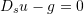is the imposed boundary conditions on the boundary, then, the residual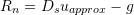is first defined and the boundary condition is incorporated by setting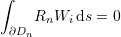Alternatively, the non-essential boundary conditions are incorporated directly in Equation 1 using integration by parts as will be shown later.

### The Weighted Residuals Methods

Assuming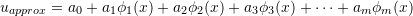, where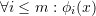is a known function and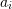is an unknown parameters, the weighted residuals methods vary in the choice of the weight functions. The following is a list of possible choices for:

#### Least Squares Method

In the least squares method, the weight functionsis chosen such that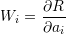The least squares method is effectively minimizing the squares of the residuals: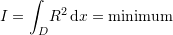#### Point Collocation Method

The Point Collocation Method described before is a special case wherepoints are chosen such that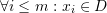and the weight functions are chosen such that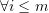: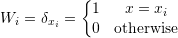#### Sub-domain Method

In this method,sub-domains are chosen such that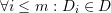and the weight functions are chosen such that: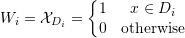#### Galerkin Method

In the Galerkin method, the weight functionsis chosen such that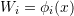The Galerkin method is effectively the virtual work method.

### Example

Using a polynomial trial function of the third degree, find the displacement function of the shown bar using the Galerkin method. Assume that the bar is linear elastic with Young’s modulus E and cross-sectional area A and that the small strain tensor is the appropriate measure of strain. Ignore the effect of Poisson’s ratio.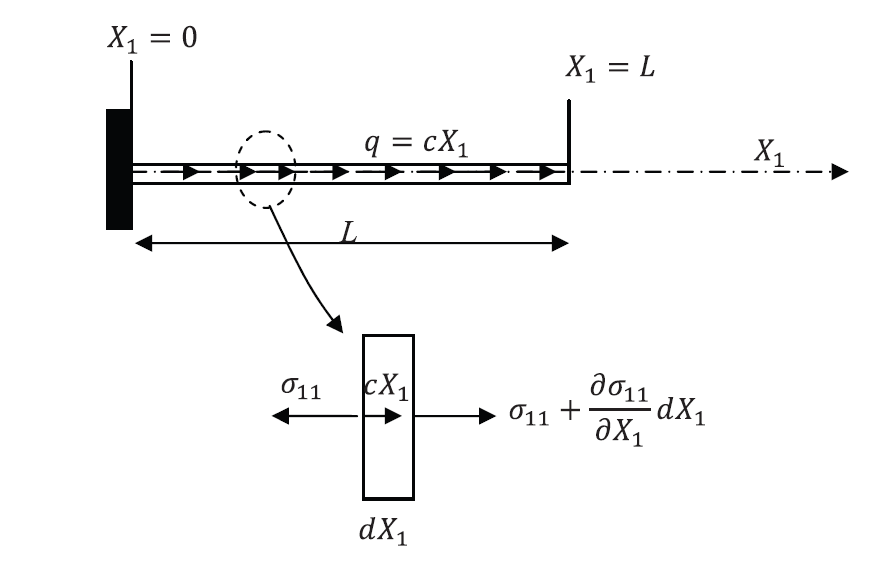##### Solution
###### Exact Solution

The exact solution can be obtained by directly solving the differential equation of equilibrium utilizing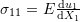: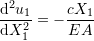with the boundary conditions: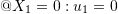and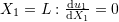.
Therefore: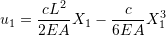View Mathematica Code
DSolve[{u''[X1] == -c*X1/EA, u'[L] == 0, u == 0}, u[X1], X1]

###### Approximation Solution

The approximate solution has the form: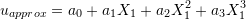There is one essential boundary condition which is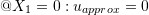, therefore,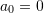.
The approximate solution thus has the following form which satisfies the essential boundary conditions: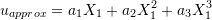where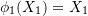,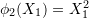, and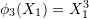are the known functions and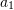,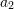, and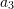are the unknown parameters. The residuals have the form: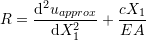The Galerkin method aims at setting the weighted residuals to zero: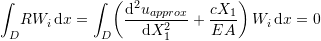Rearranging: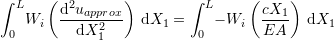Integration by parts of the left hand side can be used to incorporate the nonessential boundary conditions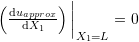as follows: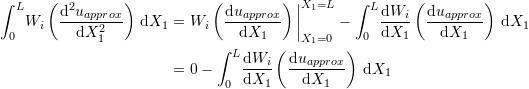Therefore, the Galerkin method equations now have the form: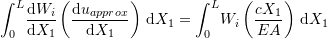To find the unknowns,, and, three equations are formed with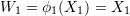,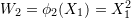, and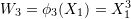. Solving those three equations the following approximate solution is obtained: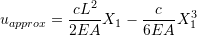Notice that since the exact solution (a polynomial of the third degree) is an element of the space of possible approximate solutions, the Galerkin method yields the exact solution!

View Mathematica Code
w1=x1;
w2=x1^2;
w3=x1^3;
u=a1*w1+a2*w2+a3*w3;
Eq1=Integrate[D[w1,x1]*D[u,x1],{x1,0,L}]-Integrate[w1*c*x1/EA,{x1,0,L}];
Eq2=Integrate[D[w2,x1]*D[u,x1],{x1,0,L}]-Integrate[w2*c*x1/EA,{x1,0,L}];
Eq3=Integrate[D[w3,x1]*D[u,x1],{x1,0,L}]-Integrate[w3*c*x1/EA,{x1,0,L}];
s=Solve[{Eq1==0,Eq2==0,Eq3==},{a1,a2,a3}]
u/.s[]


### Problems

Solve the problems in the Rayleigh Ritz method section using the Galerkin Method.